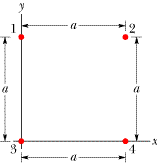# Problem: In the figure below, the particles have charges q1 = -q2 = 264 nC and q3 = -q4 = 168 nC, and distance a = 5.2 cm.What are the (a)x and (b) y components of the net electrostatic force on particle 3?

###### FREE Expert Solution

Coulomb's law:

$\overline{){\mathbf{F}}{\mathbf{=}}\frac{\mathbf{k}{\mathbf{q}}_{\mathbf{1}}{\mathbf{q}}_{\mathbf{2}}}{{\mathbf{r}}^{\mathbf{2}}}}$###### Problem Details

In the figure below, the particles have charges q1 = -q2 = 264 nC and q3 = -q4 = 168 nC, and distance a = 5.2 cm.

What are the (a)x and (b) y components of the net electrostatic force on particle 3?